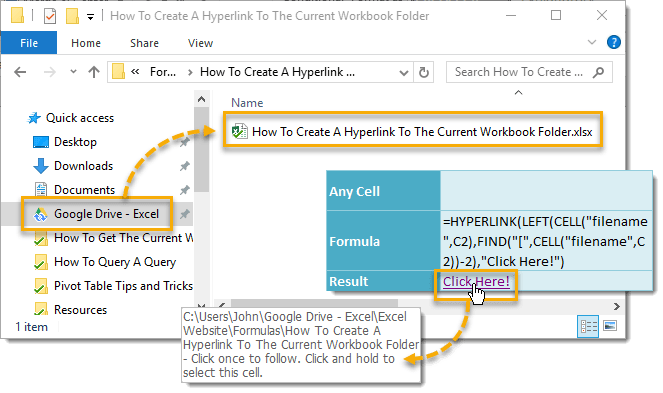## Example

``` =HYPERLINK(LEFT(CELL("filename",C2),FIND("[",CELL("filename",C2))-2),"Click Here!") ```

## Generic Formula

``` =HYPERLINK(LEFT(CELL("filename",AnyCell),FIND("[",CELL("filename",AnyCell))-2),"Text") ```

• filename” – This is a predefined Excel parameter input for the CELL function.
• AnyCell – This can be any cell in any sheet of the workbook.
• Text – This is the text you want to show instead of the folder path. If left blank the folder path will show in the hyperlink.

## What It Does

This formula will create a hyperlink that opens a file explorer window to the folder location where the current workbook is saved.

## How It Works

LEFT(CELL(“filename”,C2),FIND(“[“,CELL(“filename”,C2))-2) will return the folder path of the current workbook. See how this part of the formula works from another post. In this example, this part of the formula returns “C:\Users\John\Google Drive – Excel\Excel Website\Formulas\How To Create A Hyperlink To The Current Workbook Folder“.

HYPERLINK(Folder,”Click Here!”) then creates a hyperlink to the Folder and displays “Click Here!” in the cell. If you click the cell once a file explorer window will open at the Folder location. Click and hold to select the cell.